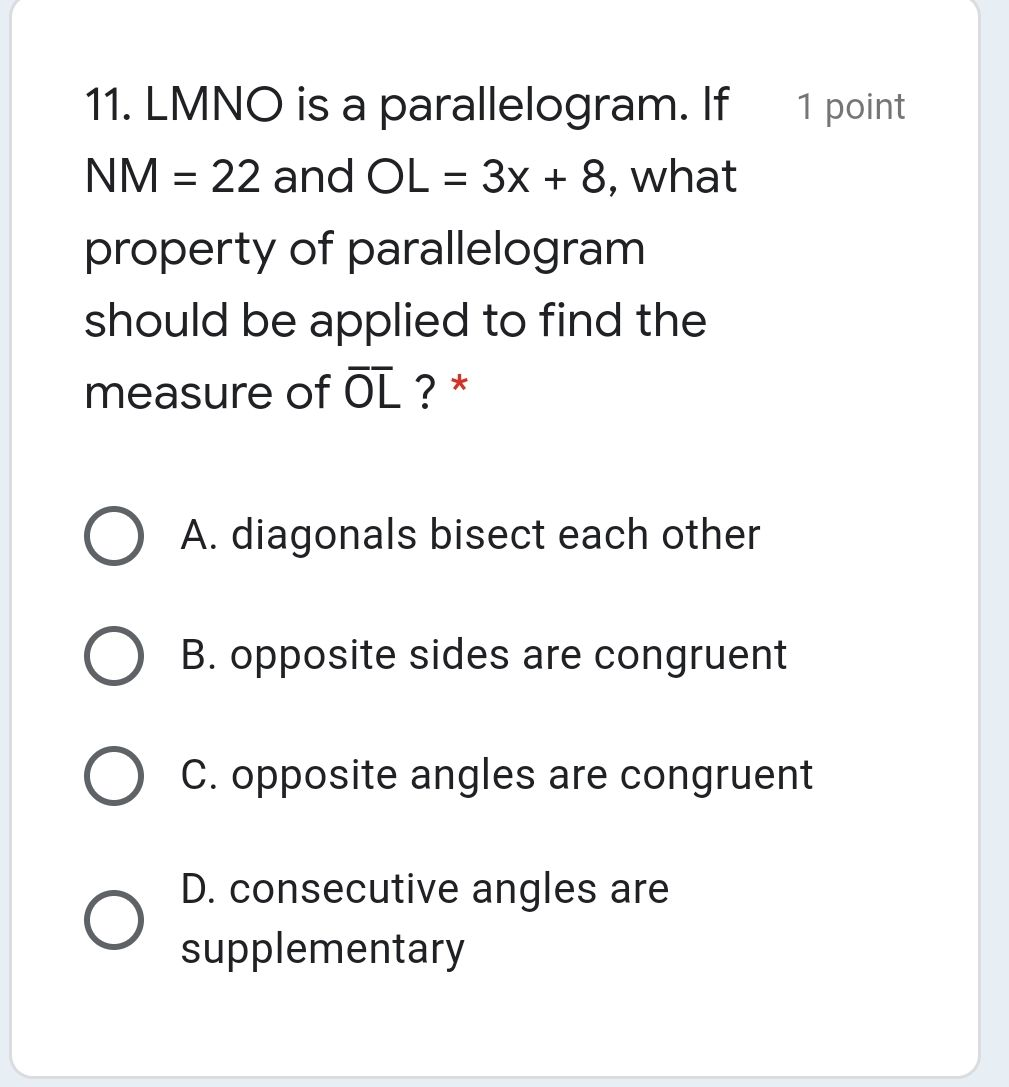### ¿Todavía tienes preguntas de matemáticas?

Pregunte a nuestros tutores expertos
Algebra
Pregunta11. LMNO is a parallelogram. If $$N M = 22$$ and $$O L = 3 x + 8$$ , what property of parallelogram should be applied to find the measure of $$\overline { O } \overline { L } ? { } ^ { * }$$

A. diagonals bisect each other

B. opposite sides are congruent

C. opposite angles are congruent

D. consecutive angles are supplementary